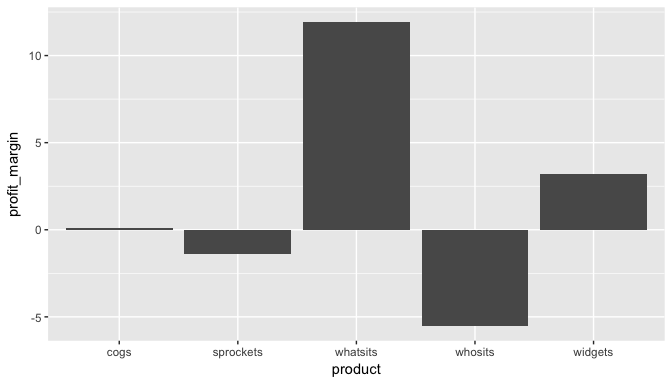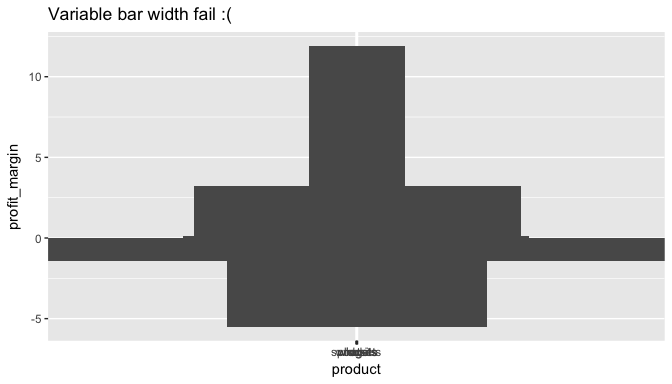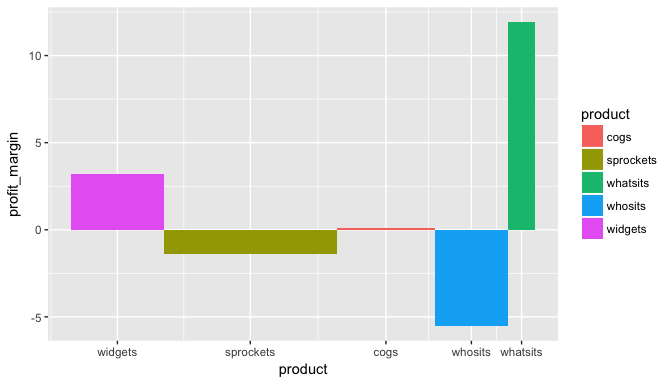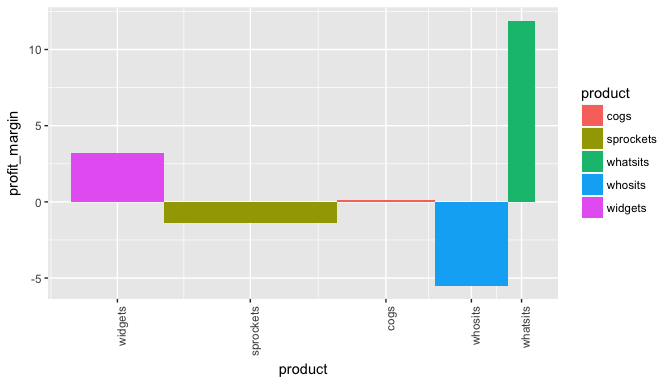# Introduction to the mekko package

### Bar mekko graphics in R

This is a quick introduction to the bar mekko function* in the mekko package.

Its main value is to add quantitative context to a bar graph, via bar width.

Install with:

install.packages("mekko")

You can also install the development version with devtools::install_github('ryninho/mekko', build_vignettes = TRUE).

View examples with:

vignette("mekko-vignette")

First we’ll load ggplot and mekko and create some example data.

library(ggplot2)
library(mekko)
profits <- data.frame(
product = c('widgets', 'sprockets', 'cogs', 'whosits', 'whatsits'),
profit_margin = c(3.2, -1.4, 0.1, -5.5, 11.9),
revenue = c(850, 1600, 900, 675, 250)
)

### Bar mekko

Now let’s take a look at profit margin by product using ggplot2.

ggplot(profits, aes(x = product, y = profit_margin)) +
geom_bar(stat = "identity")Well that’s insightful, but I don’t know how worried I should be about the margin on whosits or cogs, nor do I know how happy I should be about whatsits knocking it out of the park. Maybe I can add revenue as the bar width so I know what’s important here?

ggplot(profits, aes(x = product, y = profit_margin, width = revenue)) +
geom_bar(stat = "identity") +
labs(title = "Variable bar width fail :(")
## Warning: position_stack requires non-overlapping x intervals

## Warning: position_stack requires non-overlapping x intervalsWell shucks, that looks like some kind of Atari graphics airplane. Let’s use the mekko package to put our margins in context.

bmx <- barmekko(profits, product, profit_margin, revenue)
bmxAlright, so actually the weak profit margins on sprockets may be worth nearly as much focus as the problem with whosits. Also, no high-fives for margins on the whatsits until we triple sales of them.

Those labels are a little close together- this is a ggplot object so let’s use the usual method of rotating the axes.

bmx + theme(axis.text.x = element_text(angle = 90, hjust = 1))### Appendix

• This package originally included a marimekko function as well, but the latter was discovered to be well covered in other packages, so it was removed to simplify maintenance
• While the barmekko is a ggplot object, your mileage may vary when attempting operations that normally would work on a ggplot object, as the data frame is deconstructed in the process of creating the graph